# Create a calendar

This post will guide you how to create a monthly calendar with VBA code in Excel. How do I create a calendar with calendar template in excel. How to create a yearly calendar in Microsoft Excel.

## Create a Yearly Calendar

You can use the Calendar template to create a yearly calendar, just do the following steps:

#1 click File tab, and select the New menu from the pop-up menu list. The New page will be displayed in the right of the window.

#2 enter calendar string in the search text box in New page. Then press Enter key in your keyboard.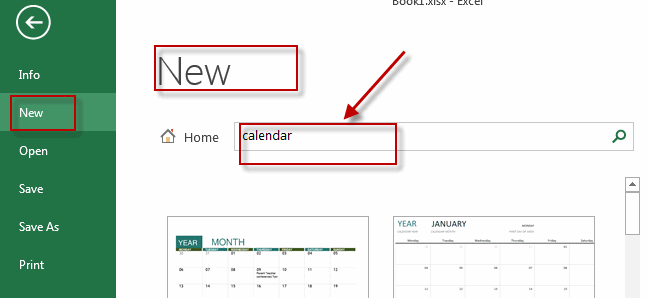#3 select one calendar template that you want to create, and then click Create button.

#4 Then the selected template will be downloaded. And a new workbook that contain calendar sheet will be created.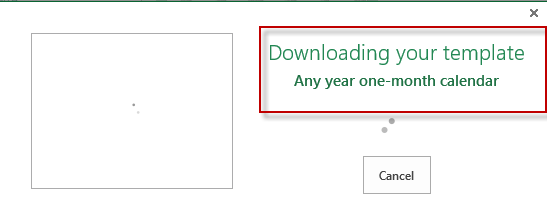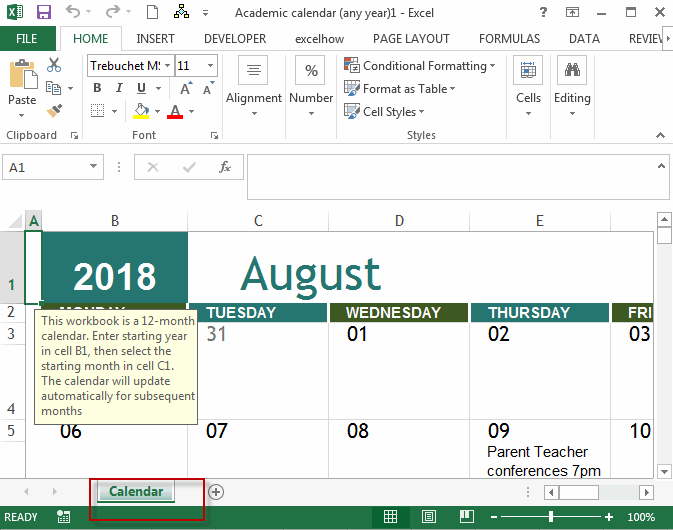## Create a Monthly Calendar

You can also use an Excel VBA macro to create a monthly calendar quickly in your worksheet. You just need to do the following steps:

#1 open your excel workbook and then click on “Visual Basic” command under DEVELOPER Tab, or just press “ALT+F11” shortcut.#2 then the “Visual Basic Editor” window will appear.

#3 click “Insert” ->”Module” to create a new module#4 paste the below VBA code into the code window. Then clicking “Save” button.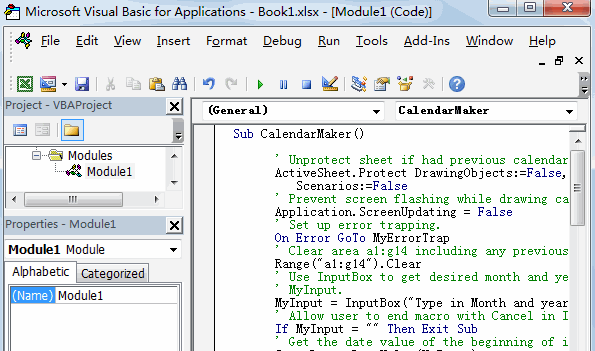``` Sub CalendarMaker()

' Unprotect sheet if had previous calendar to prevent error.
ActiveSheet.Protect DrawingObjects:=False, Contents:=False, _
Scenarios:=False
' Prevent screen flashing while drawing calendar.
Application.ScreenUpdating = False
' Set up error trapping.
On Error GoTo MyErrorTrap
' Clear area a1:g14 including any previous calendar.
Range("a1:g14").Clear
' Use InputBox to get desired month and year and set variable
' MyInput.
MyInput = InputBox("Type in Month and year for Calendar ")
' Allow user to end macro with Cancel in InputBox.
If MyInput = "" Then Exit Sub
' Get the date value of the beginning of inputted month.
StartDay = DateValue(MyInput)
' Check if valid date but not the first of the month
' -- if so, reset StartDay to first day of month.
If Day(StartDay) <> 1 Then
StartDay = DateValue(Month(StartDay) & "/1/" & _
Year(StartDay))
End If
' Prepare cell for Month and Year as fully spelled out.
Range("a1").NumberFormat = "mmmm yyyy"
' Center the Month and Year label across a1:g1 with appropriate
' size, height and bolding.
With Range("a1:g1")
.HorizontalAlignment = xlCenterAcrossSelection
.VerticalAlignment = xlCenter
.Font.Size = 18
.Font.Bold = True
.RowHeight = 35
End With
' Prepare a2:g2 for day of week labels with centering, size,
' height and bolding.
With Range("a2:g2")
.ColumnWidth = 11
.VerticalAlignment = xlCenter
.HorizontalAlignment = xlCenter
.VerticalAlignment = xlCenter
.Orientation = xlHorizontal
.Font.Size = 12
.Font.Bold = True
.RowHeight = 20
End With
' Put days of week in a2:g2.
Range("a2") = "Sunday"
Range("b2") = "Monday"
Range("c2") = "Tuesday"
Range("d2") = "Wednesday"
Range("e2") = "Thursday"
Range("f2") = "Friday"
Range("g2") = "Saturday"
' Prepare a3:g7 for dates with left/top alignment, size, height
' and bolding.
With Range("a3:g8")
.HorizontalAlignment = xlRight
.VerticalAlignment = xlTop
.Font.Size = 18
.Font.Bold = True
.RowHeight = 21
End With
' Put inputted month and year fully spelling out into "a1".
Range("a1").Value = Application.Text(MyInput, "mmmm yyyy")
' Set variable and get which day of the week the month starts.
DayofWeek = Weekday(StartDay)
' Set variables to identify the year and month as separate
' variables.
CurYear = Year(StartDay)
CurMonth = Month(StartDay)
' Set variable and calculate the first day of the next month.
FinalDay = DateSerial(CurYear, CurMonth + 1, 1)
' Place a "1" in cell position of the first day of the chosen
' month based on DayofWeek.
Select Case DayofWeek
Case 1
Range("a3").Value = 1
Case 2
Range("b3").Value = 1
Case 3
Range("c3").Value = 1
Case 4
Range("d3").Value = 1
Case 5
Range("e3").Value = 1
Case 6
Range("f3").Value = 1
Case 7
Range("g3").Value = 1
End Select
' Loop through range a3:g8 incrementing each cell after the "1"
' cell.
For Each cell In Range("a3:g8")
RowCell = cell.Row
ColCell = cell.Column
' Do if "1" is in first column.
If cell.Column = 1 And cell.Row = 3 Then
' Do if current cell is not in 1st column.
ElseIf cell.Column <> 1 Then
If cell.Offset(0, -1).Value >= 1 Then
cell.Value = cell.Offset(0, -1).Value + 1
' Stop when the last day of the month has been
' entered.
If cell.Value > (FinalDay - StartDay) Then
cell.Value = ""
' Exit loop when calendar has correct number of
' days shown.
Exit For
End If
End If
' Do only if current cell is not in Row 3 and is in Column 1.
ElseIf cell.Row > 3 And cell.Column = 1 Then
cell.Value = cell.Offset(-1, 6).Value + 1
' Stop when the last day of the month has been entered.
If cell.Value > (FinalDay - StartDay) Then
cell.Value = ""
' Exit loop when calendar has correct number of days
' shown.
Exit For
End If
End If
Next

' Create Entry cells, format them centered, wrap text, and border
' around days.
For x = 0 To 5
Range("A4").Offset(x * 2, 0).EntireRow.Insert
With Range("A4:G4").Offset(x * 2, 0)
.RowHeight = 65
.HorizontalAlignment = xlCenter
.VerticalAlignment = xlTop
.WrapText = True
.Font.Size = 10
.Font.Bold = False
' Unlock these cells to be able to enter text later after
' sheet is protected.
.Locked = False
End With
' Put border around the block of dates.
With Range("A3").Offset(x * 2, 0).Resize(2, _
7).Borders(xlLeft)
.Weight = xlThick
.ColorIndex = xlAutomatic
End With

With Range("A3").Offset(x * 2, 0).Resize(2, _
7).Borders(xlRight)
.Weight = xlThick
.ColorIndex = xlAutomatic
End With
Range("A3").Offset(x * 2, 0).Resize(2, 7).BorderAround _
Weight:=xlThick, ColorIndex:=xlAutomatic
Next
If Range("A13").Value = "" Then Range("A13").Offset(0, 0) _
.Resize(2, 8).EntireRow.Delete
' Turn off gridlines.
ActiveWindow.DisplayGridlines = False
' Protect sheet to prevent overwriting the dates.
ActiveSheet.Protect DrawingObjects:=True, Contents:=True, _
Scenarios:=True

' Resize window to show all of calendar (may have to be adjusted
' for video configuration).
ActiveWindow.WindowState = xlMaximized
ActiveWindow.ScrollRow = 1

' Allow screen to redraw with calendar showing.
Application.ScreenUpdating = True
' Prevent going to error trap unless error found by exiting Sub
' here.
Exit Sub
' Error causes msgbox to indicate the problem, provides new input box,
' and resumes at the line that caused the error.
MyErrorTrap:
MsgBox "You may not have entered your Month and Year correctly." _
& Chr(13) & "Spell the Month correctly" _
& " (or use 3 letter abbreviation)" _
& Chr(13) & "and 4 digits for the Year"
MyInput = InputBox("Type in Month and year for Calendar")
If MyInput = "" Then Exit Sub
Resume
End Sub```

The above Code is from Microsoft official site.

#5 back to the current worksheet, then run the above excel macro. Click Run button.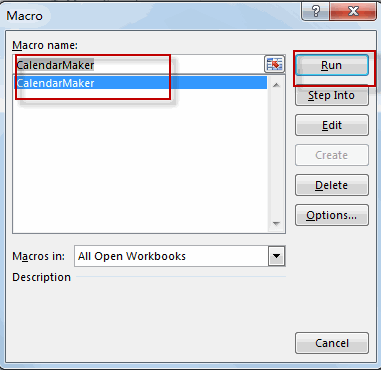#6 type in Month and year for calendar, and then click OK button.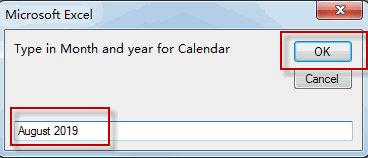#7 the Monthly calendar is created in your current worksheet.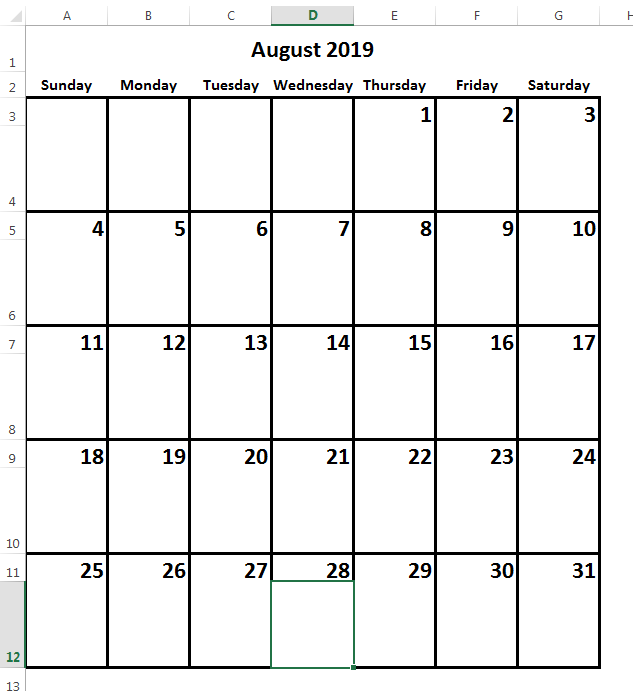### Related posts

Create Drop Down Calendar

Related Posts

Find and Replace Multiple Values

This post will guide you how to find and replace multiple values at once with VBA macro or using formula  in Excel. How do I make multiple find and replace in Excel. Suppose that you have a few cells containing ...

VBA Macro For VLOOKUP From Another Sheet

In the previous post, you should know that how to fix or remove the #N/A error when using VLOOKUP formula to lookup value from another sheet. And this post will show you how to use VBA code to vlookup data ...

How To Insert Comments in Protected Worksheet in Excel

This post will show you how to allow comments in a protected worksheet in Excel. You can easily to insert comments into cells in a normal worksheet in Excel, but if want to insert a comment in a worksheet that ...

How To Convert Text to Upper Cases(Using VBA) in Excel

This post will show you how to switch from lower case to upper case in Excel. and I am going to show you two different ways of converting text to upper cases using formula or VBA macro in Excel 2013,Excel ...

How To Hide Every Other Row in Excel (Using VBA)

This post will show you how to hide alternate rows or columns in Excel or how to hide every third, fourth, fifth row or column in Excel. If you want to hide every other row in your current worksheet, how ...

How to Disable the Save As Prompt in Excel

This post will show you how to use a VBA Macro to save an Excel file and overwrite any existing file without a prompt so that you are going to get the little window that says file already exists do ...

How to Count Cells that Contain even or odd numbers in Excel

This post will guide you how to count the number of cells that contain odd or even numbers within a range of cells using a formula in Excel 2013/2016.How do I count cells that contain odd numbers through the use ...

How to Count Cells that Contain negative Numbers in Excel

This post will guide you how to count the number of cells that contain negative numbers within a range of cells using a formula in Excel 2013/2016.You can count the number of negative numbers in your data using easy functions ...

How to Count Cells Are Not Blank or Empty in Excel

This post will guide you how to count cells that are not blank or empty in a given range cells using a formula in Excel 2013/2016.How do I count the number of cells that are not blank in a particular ...

How to Count Cells Less Than a Specific Value in Excel

This post will guide you how to count the number of cells less than a particular numeric value in a given range cells using a formula in Excel 2013/2016. How do I count cells that are less than a specific ...

Sidebar